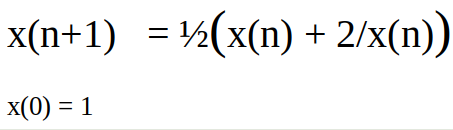# Math Contest #20 Results and Solution## Solution

The problem of this contest was to find the limit of a sequence:1. `Does this series converge?`
Yes: This sequence returns the average of x and 2/x in each iteration. And since x will always stay bigger than one this will slowly reduce the difference between the two until an equillibrium is reached.
2. `What is the limit?`
For x → ∞ the difference between two consecutive members of the sequence will go to zero, so for x → ∞:
`x(n+1) = x(n) = ½(x(n) + 2/x(n))`
`2x(n) = x(n) + 2/x(n)`
`2x(n)² = x(n)² + 2`
`x(n)² = 2`

`x = √2`
3. `Bonus: Construct a similar sequence for another root`
This can be done by reversing above steps:
`x = √a`

`x(n)² = a`
`2x(n)² = x(n)² + a`
`2x(n) = x(n) + a/x(n)`
`x(n) = ½(x(n) + a/x(n))`

`x(n+1) = ½(x(n) + a/x(n))` is a sequence that converges to √a.

↓↑↓↑↓↑↓↑↓↑↓↑↓↑↓↑↓↑↓↑↓↑↓↑↓↑↓↑↓↑↓↑↓↑↓↑↓↑↓↑↓↑↓↑↓↑↓↑↓

### List of participants with their entries:

Name solutions found comment
@crokkon all correct +bonus
@kaeserotor all correct +bonus

↓↑↓↑↓↑↓↑↓↑↓↑↓↑↓↑↓↑↓↑↓↑↓↑↓↑↓↑↓↑↓↑↓↑↓↑↓↑↓↑↓↑↓↑↓↑↓↑↓

## Winner draw:

2 SBI for 2 person → you both won 1 SBI and 10 STEM each!
↓↑↓↑↓↑↓↑↓↑↓↑↓↑↓↑↓↑↓↑↓↑↓↑↓↑↓↑↓↑↓↑↓↑↓↑↓↑↓↑↓↑↓↑↓↑↓↑↓